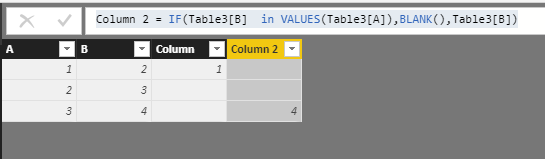cancel
Showing results for
Did you mean:Frequent Visitor

## From R to Power Bi - how to use setdiff

I've got working script in R

Two data frames: zerowy_nazwa5, zatwierdzony_nazwa5

and working 2 lines:
setdiff(zatwierdzony_nazwa5, zerowy_nazwa5)
setdiff(zerowy_nazwa5, zatwierdzony_nazwa5)

how i implement this in PowerBi?

1 ACCEPTED SOLUTIONCommunity Support

Hi@ Waldek

After my research,You may try to do these follow my steps like below:

Step 1:

```Column = IF(Table3[A]  in VALUES(Table3[B]),BLANK(),Table3[A])
Column 2 = IF(Table3[B]  in VALUES(Table3[A]),BLANK(),Table3[B])```Step 2:

`Table 2 = FILTER(UNION(VALUES(Table3[Column]),VALUES(Table3[Column 2])),ISBLANK(Table3[Column] )=FALSE())`

Result:Best Regards,

Lin

Community Support Team _ Lin
If this post helps, then please consider Accept it as the solution to help the other members find it more quickly.Community Support

Hi@ Waldek

After my research,You may try to do these follow my steps like below:

Step 1:

```Column = IF(Table3[A]  in VALUES(Table3[B]),BLANK(),Table3[A])
Column 2 = IF(Table3[B]  in VALUES(Table3[A]),BLANK(),Table3[B])```Step 2:

`Table 2 = FILTER(UNION(VALUES(Table3[Column]),VALUES(Table3[Column 2])),ISBLANK(Table3[Column] )=FALSE())`

Result:Best Regards,

Lin

Community Support Team _ Lin
If this post helps, then please consider Accept it as the solution to help the other members find it more quickly.